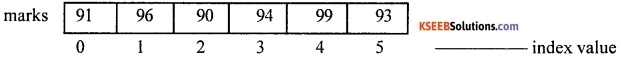# 1st PUC Computer Science Question Bank Chapter 11 Arrays

## Karnataka 1st PUC Computer Science Question Bank Chapter 11 Arrays

### 1st PUC Computer Science Arrays One Mark Questions and Answers

Question 1.
What is an Array?
Array is a group of similar elements that share a common name.

Question 2.
What is the use of arrays?
The use of arrays reduces the creation and use of more number of individual variables in a program. It also helps to reduce the program length.

Question 3.
Mention the different types of arrays.
There are three types of arrays, namely

1. One dimensional array,
2. Two-dimensional array and
3. Multidimensional arrayQuestion 4.
What is index or subscript of an Array?
Subscript is used to identify/locate the position of array elements.

Question 5.
What is zero-based indexing?
If ‘i’ is the element, then its index number would be i-1 and it is called zero-based indexing.

Question 6.
What is the subscript of the first element of an array?
The first element i = 1, then subscript is 0 because of i-1 i.e., 1-1

Question 7.
Mention any one feature of subscript or index of an array.
Subscript or index of an array is always a positive whole number.

Question 8.
Define a one-dimensional array.
One dimensional array is a collection of similar elements that share a common name and is structured in one dimension only.

Question 9.
Give the declaration syntax of a one-dimensional array.
datatype array name [size];

Question 10.
What is meant by initialization of an array?
Initialization means assigning values to the declared variables or arrays. To initialize an array, each and every element of the array has to be referred and then value assigned to it.Question 11.
Give an example showing initialization of one-dimensional array.
int marks = { 91,96,90,94,99,93};

Question 12.
Define a two-dimensional array.
Two-dimensional array is a collection of elements of similar type that share a common name, structured in two dimensions. Each element is accessed by two index values.

Question 13.
Write the declaration syntax of a two-dimensional array.
Syntax:
<data-type> <array-name> [<size1>] [<size2>];

Question 14.
Give an example of, how a two-dimensional array is declared?
int marks;

Question 15.
Give one example showing initialization of a two-dimensional array.
Example of array initialization with declaration:
int matrix   = { 91,96,90,94,99,95,87,92,98};

Question 16.
Declare an array to store the 6 subject marks of 500 students.
Int marks  ;Question 17.
How an element in the two-dimensional array is accessed?
An element in the two-dimensional array is accessed using two index/subscript numbers. The first number indicates the row index and the second number indicates the column index.

Question 18.
Define multidimensional array.
A collection of similar elements structured in N dimensions where N>=1. Each element is accessed by N indices.

### 1st PUC Computer Science Arrays Two/ Three Marks Questions and Answers

Question 1.
Explain any two uses of arrays.
It reduces the creation and use of more number of individual variables in a program. Confusion and complexity in identifying the variables can be avoided.

Question 2.
Write any two features of an array.
Array can be of any data type available in ‘C++’, i.e., integer array, float array, character array, etc., All the elements of an array will contain the same type of data.

Question 3.
What are the characteristics of index/subscript of any array?
It is always a positive whole number. It may be an integer, constant or expression and cannot be a negative integer or floating value. The first element of an array always starts with the value 0.

Question 4.
Give an explanation of the declaration of one-dimensional array with an example.
int marks;
Name of the array is marks.
Type of the array is integer.
Size of the array is 6. i.e., we can store upto 6 integer values in the array.

Question 5.
Write the array representation of the initialization of a one-dimensional array with an example.
int marks = { 91,96,90,94,99,93};Question 6.
How can we input the values into a one-dimensional array?
Using ‘for’ loop:
int age , i;
for(i=0; i<10; i++)
{
cin>>age[i];
}Question 7.
How are elements of one-dimensional array displayed?
for(i=0;i<10;i++)
cout<<age[i];

Question 8.
Give an explanation of the declaration of a two-dimensional array with an example.
int marks;

1. Name of the array is marks.
2. Type of the array is integer.
3. Size of the array is 5 rows and 5 columns, i.e., we can store up to 25 integer values in the array (row x column) 5 × 5 = 25.

Question 9.
Write the array representation of initialization of a two-dimensional array with an example.
int matrix   = { 91, 96, 90, 94, 99, 95, 87, 92, 98};
Two-dimensional array representation with values:Row index values

Question 10.
How can we input the values into a two-dimensional array?
int mat, row, col;
for (row = 0; row < 3; row++)
for (col = 0; col < 5; col++)
cin >> mat[row] [col];

Question 11.
Write the declaration of multidimensional array with an example.